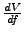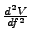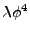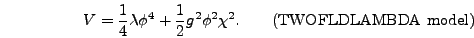Next: Writing Down the Equations Up: Using LATTICEEASY Previous: Double Precision

# Writing a New Model

Ultimately the main reason you would want to use LATTICEEASY is presumably to do lattice simulations of your own favorite models. Writing a new model for the program requires the following steps, each of which is described in more detail in a later section:

1. Write down the equations for your model. You need to specify the field content and the potential, figure out what variable rescalings to use, and then work out a few quantities likeand. These instructions tell you step by step how to write these equations in the form expected by the program.
2. Write a model file for your model. The easiest way to do this is to copy an existing model file and modify it as needed. We describe below exactly what parameters and functions the model file should contain and how to specify them correctly. Once you've written the file simply copy it into the program directory with the name model.h.
Once you have the hang of it the entire process from deciding to run a particular model to having your simulations up and going should take no more than a few hours.

The following sections go through each of these steps in detail. For illustration purposes an example will be developed as we go. We chose as our example chaotic inflation with ainflaton potential and one other field coupled to the inflaton. The potential for this model is(4.2)

The TWOFLDLAMBDA model is the model.h file in the program directory in the distribution of LATTICEEASY. To avoid confusion all formulas in this section that are specific to this model will be marked (like the preceding equation).

SubsectionsNext: Writing Down the Equations Up: Using LATTICEEASY Previous: Double Precision